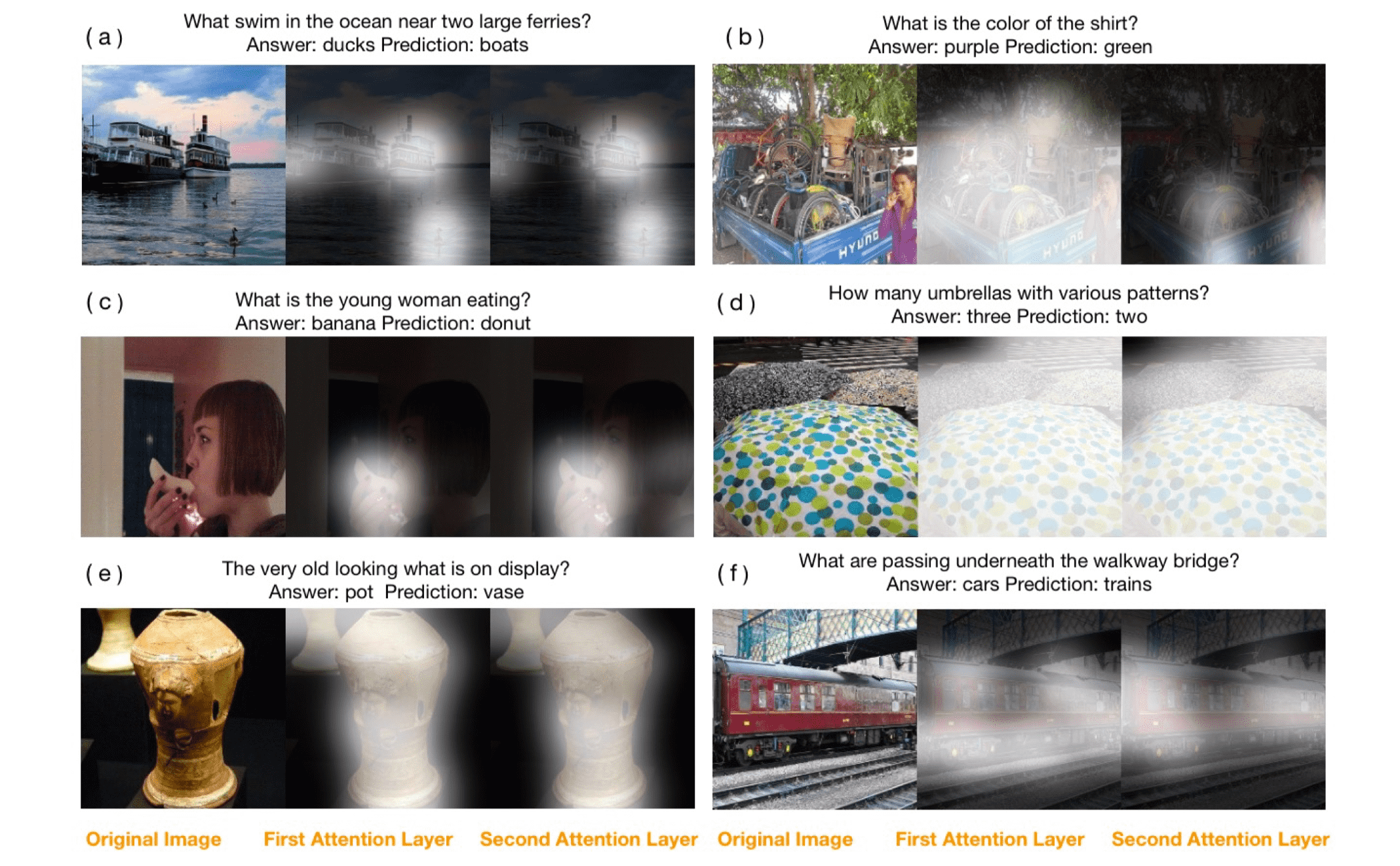# NIUHE

### Paper Reading - Stacked Attention Networks for Image QA

Zichao Yang, Xiaodong He, Jianfeng Gao , Li Deng , Alex Smola Stacked Attention Networks for Image Question Answering

SAN总共由三部分组成：

• Image Model：用来编码图片信息
• Question Moel：用来编码问题信息
• Stacked Attention Networks：通过多层 attention layer 不断优化对问题的编码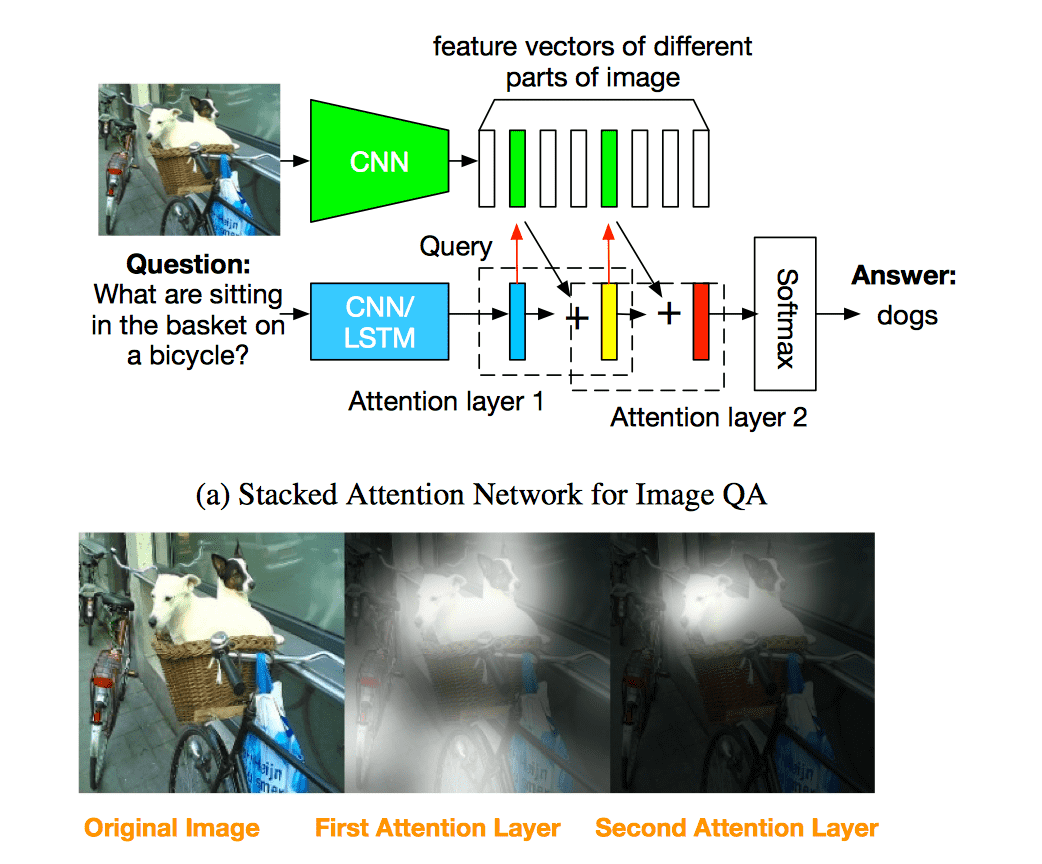## Image Model

Image model 使用 VGGNet 处理源图片，用最后一个池化层作为提取的图片特征: $f_I = CNN_{vgg}(I)$ 把输入图片转化为 $448 \times 448$ 大小， 输出的特征即为 $512 \times 14 \times 14$，其中 $512$ 为特征向量（feature vector） $f_i$ 的维度，$14 \times 14$ 是区域（特征向量）的个数，每个特征向量 $f_i$ 代表源图片中 $32 \times 32$ 大小的区域。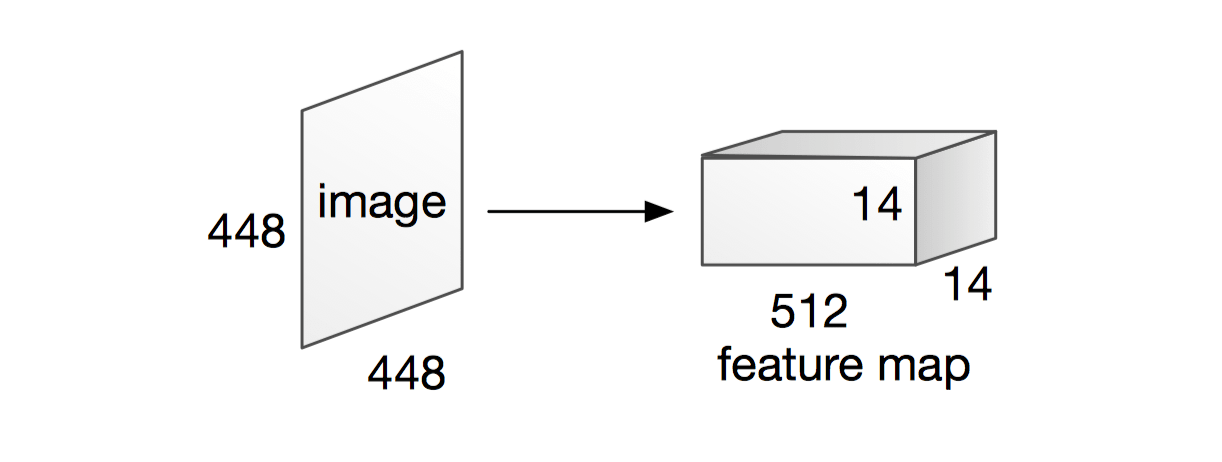## Question Model

### LSTM based question model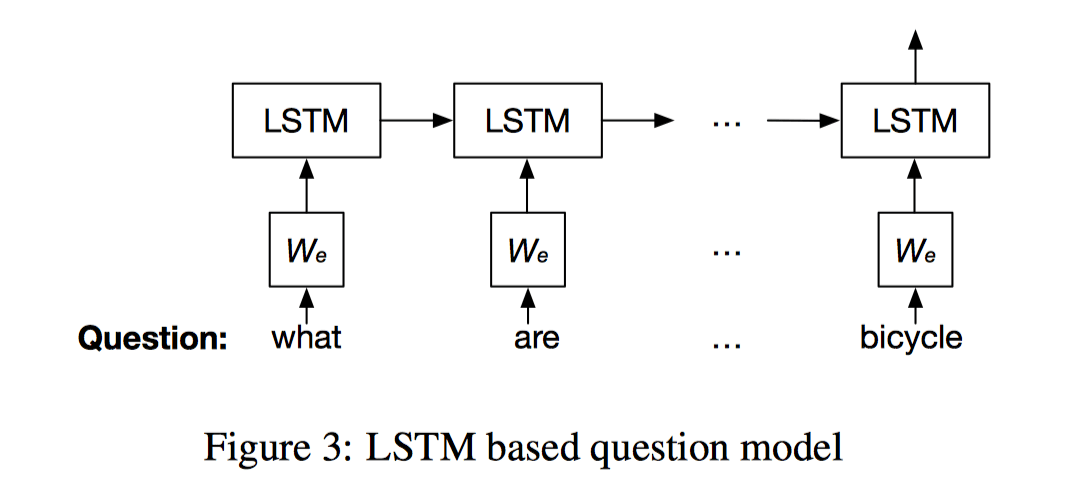### CNN based question model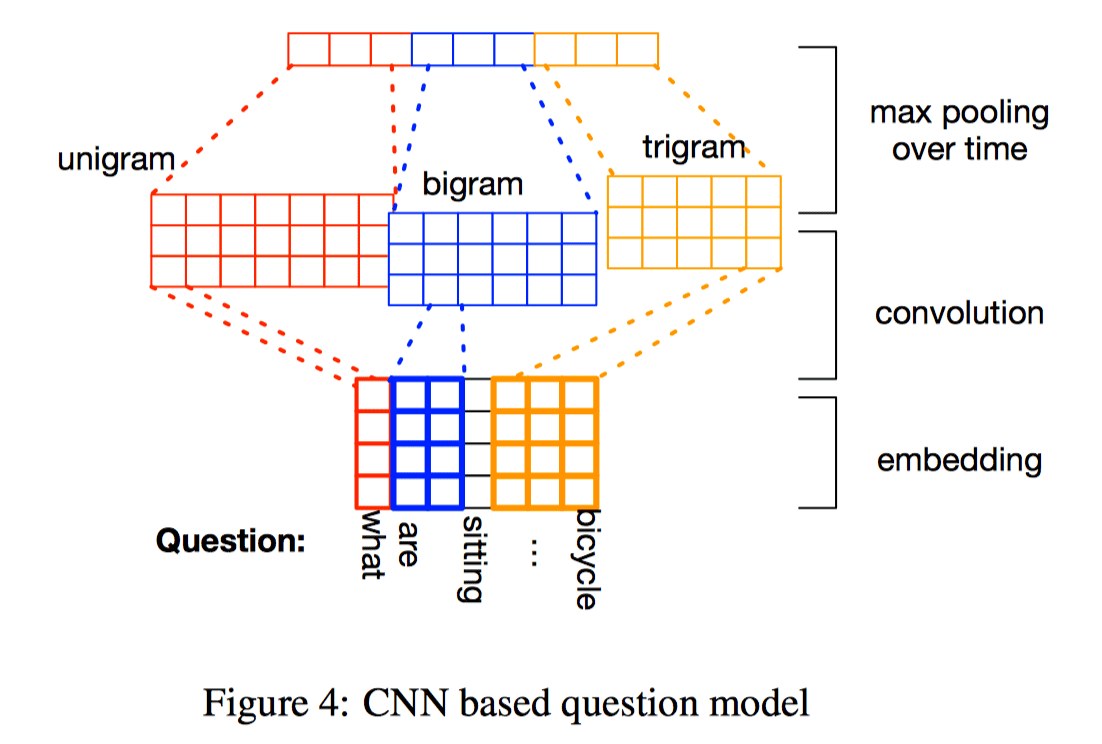$v_Q = h = [\hat{h}_1,\hat{h}_2,\hat{h}_3]$

## Stacked Attention Networks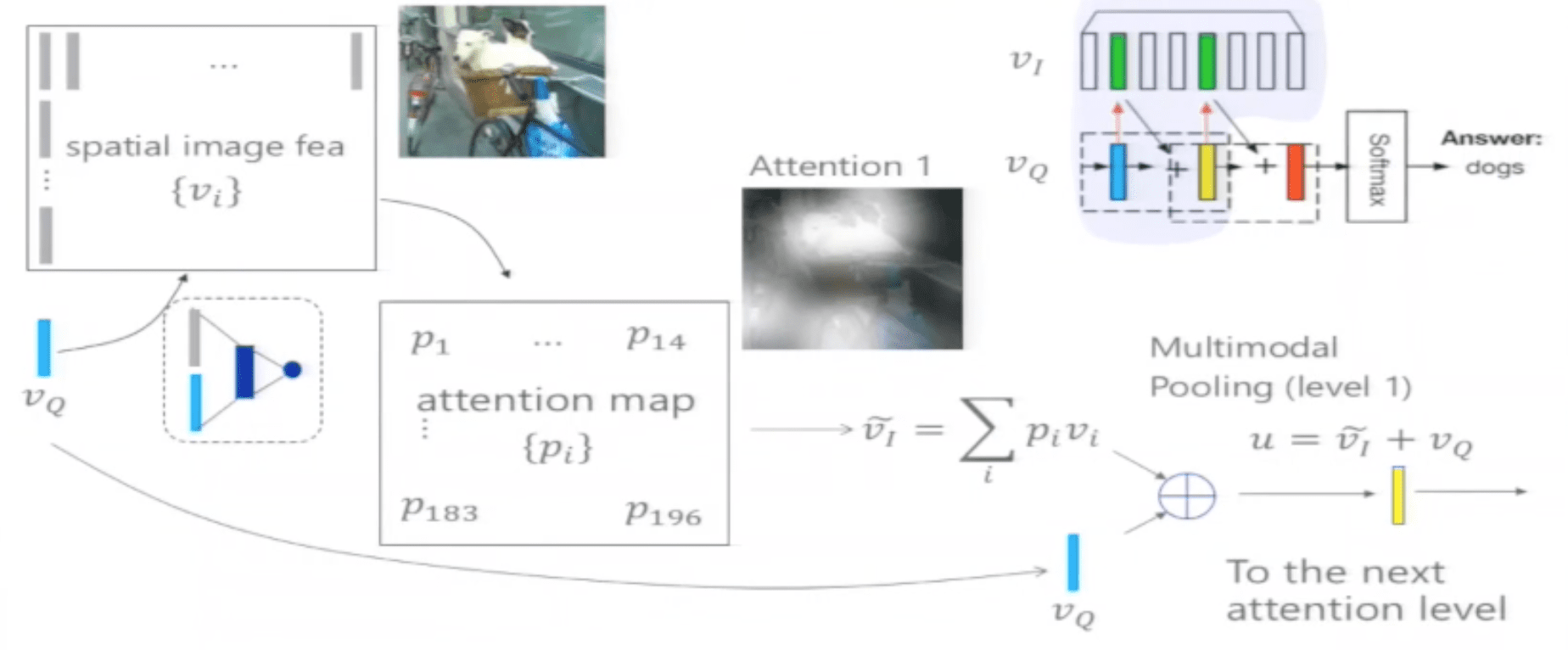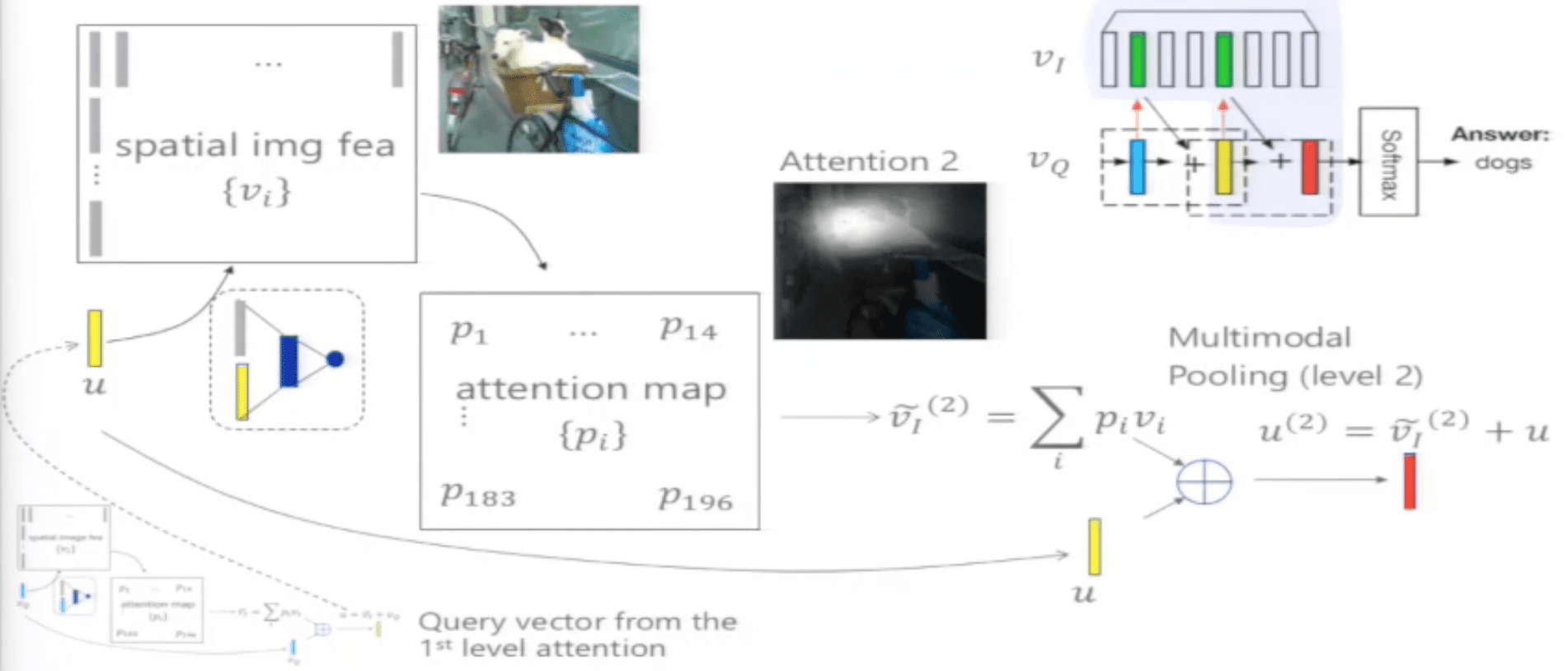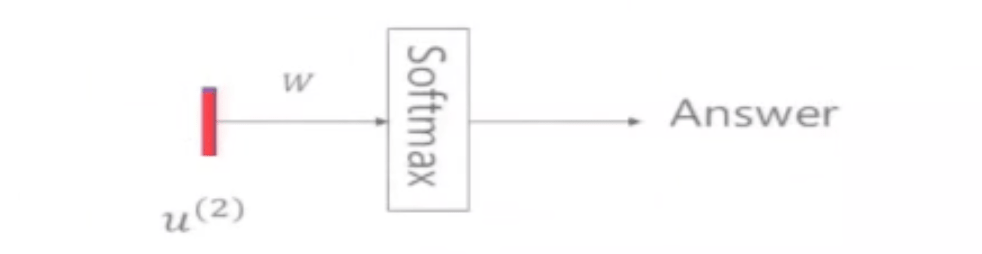## 可视化 Attention Layer

• 正确结果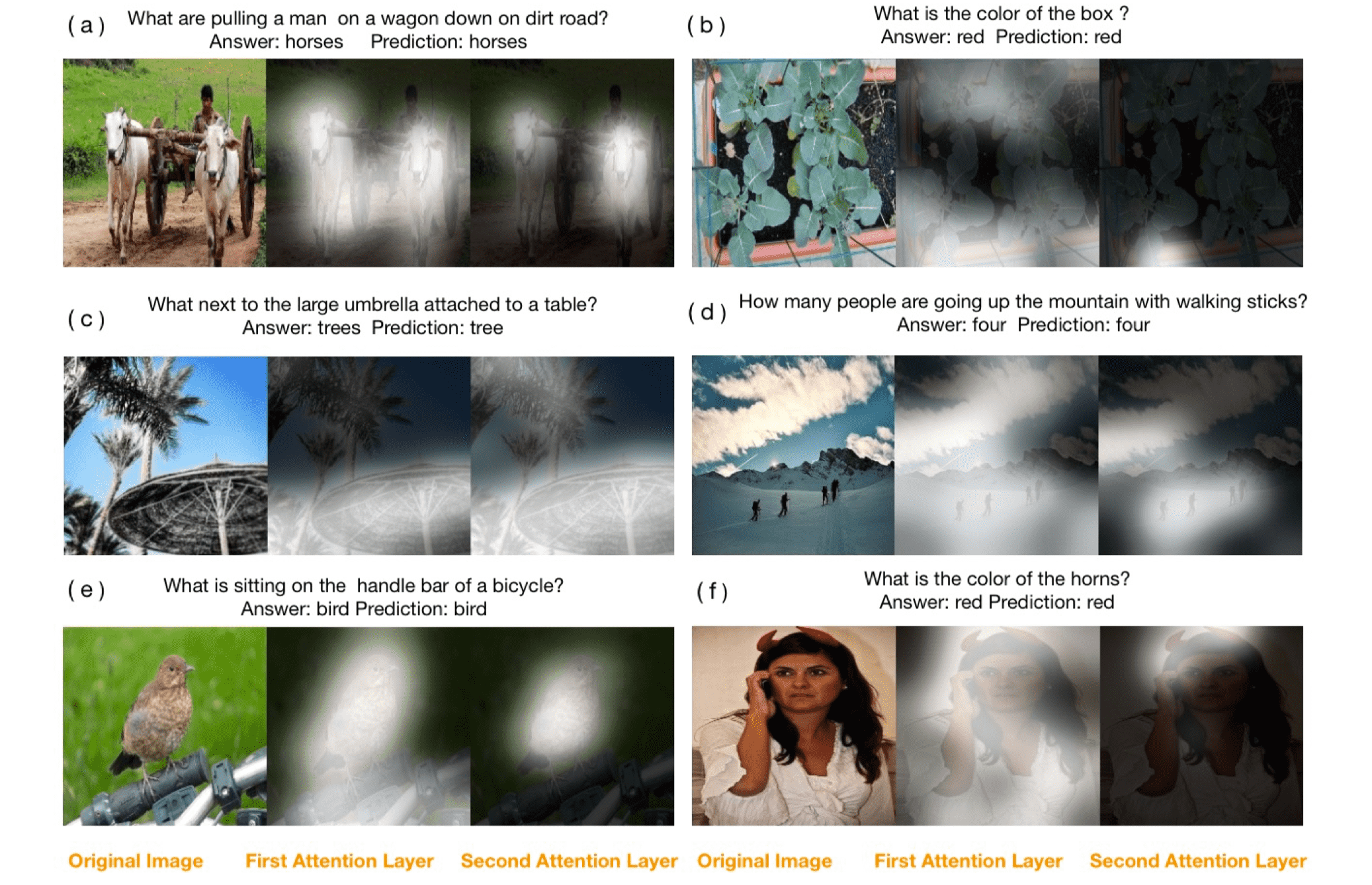• 错误结果